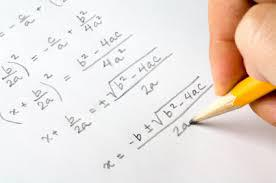# COURSE DESCRIPTIONIn Grade 8, instructional time should focus on three critical areas: (1) formulating and reasoning about expressions and equations, including modeling an association in bivariate data with a linear equation, and solving linear equations and systems of linear equations; (2) grasping the concept of a function and using functions to describe quantitative relationships; (3) analyzing two- and three-dimensional space and figures using distance, angle, similarity, and congruence, and understanding and applying the Pythagorean Theorem.

# CHAPTERS

Ch.1 – Geometry
Reflections and Translations
Transformations that Result in Congruent Figures
Transformations
Transformations Involving Dilations
Angles in Triangles
Angles and Parallel Lines
The Pythagorean Theorem
The Pythagorean Theorem and Its Converse
Distance Between Two Points
Volume of Cones, Cylinders, and Spheres
Reflections
Using the Slope and y-intercept to Write the Equation of a Line

Ch.2 – Number System
Rational and Irrational Numbers
Irrational & Rational Numbers on a Number Line
Estimating Number Locations
Factors and Divisibility
Ordering Small Numbers
Understanding Percents

Ch.3 – Expressions and Equations
Working with Square and Cube Roots
Solving Two-Step Equations with Fractions and Decimals
Multiplying and Dividing Monomials
Solve a System of Equations by Graphing
Solving Multi-Step Equations
Slope and the Equation of Lines
Proportional Linear Equations
Operations on Very Large and Very Small Numbers
Exponents: Scientific Notation
Multiplication and Division Rules for Exponents
Exponents in Expressions
Slope and the Equation of Lines
Operations on Very Large and Very Small Numbers
Dividing Polynomials by Monomials
Solving Inequalities
Slope-Intercept Form
Finding Values that Satisfy an Equation
Non-Perfect Square Roots
Graphing Inequalities